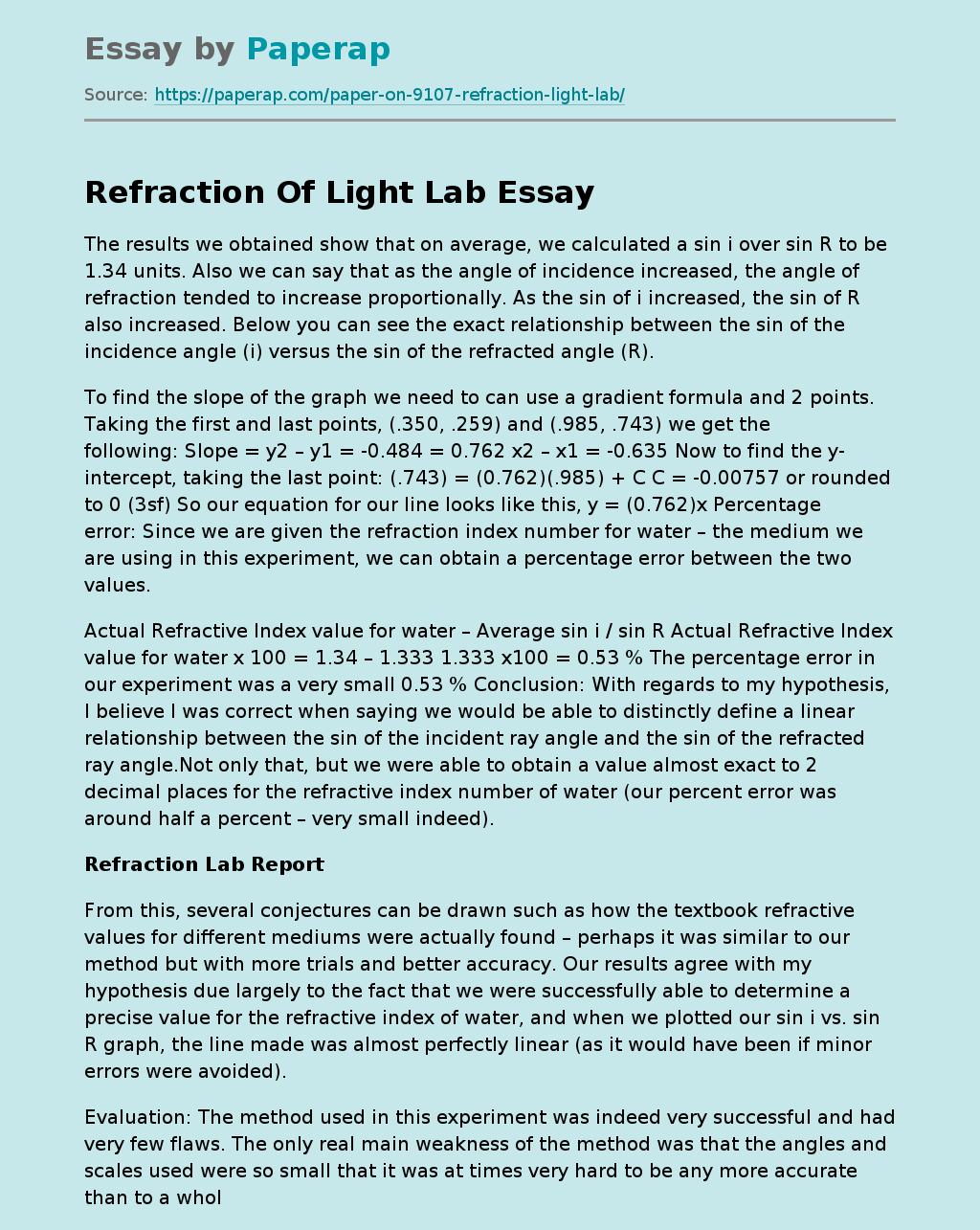# Refraction Of Light Lab

The results we obtained show that on average, we calculated a sin i over sin R to be 1.34 units. Also we can say that as the angle of incidence increased, the angle of refraction tended to increase proportionally. As the sin of i increased, the sin of R also increased. Below you can see the exact relationship between the sin of the incidence angle (i) versus the sin of the refracted angle (R).

To find the slope of the graph we need to can use a gradient formula and 2 points.

Taking the first and last points, (.350, .259) and (.985, .743) we get the following: Slope = y2 – y1 = -0.484 = 0.762 x2 – x1 = -0.635 Now to find the y-intercept, taking the last point: (.743) = (0.762)(.985) + C C = -0.00757 or rounded to 0 (3sf) So our equation for our line looks like this, y = (0.762)x Percentage error: Since we are given the refraction index number for water – the medium we are using in this experiment, we can obtain a percentage error between the two values.

Actual Refractive Index value for water – Average sin i / sin R Actual Refractive Index value for water x 100 = 1.34 – 1.333 1.333 x100 = 0.53 % The percentage error in our experiment was a very small 0.53 % Conclusion: With regards to my hypothesis, I believe I was correct when saying we would be able to distinctly define a linear relationship between the sin of the incident ray angle and the sin of the refracted ray angle.Not only that, but we were able to obtain a value almost exact to 2 decimal places for the refractive index number of water (our percent error was around half a percent – very small indeed).

Get quality help nowWriter LylaVerified

Proficient in: Data5 (876)

“ Have been using her for a while and please believe when I tell you, she never fail. Thanks Writer Lyla you are indeed awesome ”+84 relevant experts are online

## Refraction Lab Report

From this, several conjectures can be drawn such as how the textbook refractive values for different mediums were actually found – perhaps it was similar to our method but with more trials and better accuracy. Our results agree with my hypothesis due largely to the fact that we were successfully able to determine a precise value for the refractive index of water, and when we plotted our sin i vs. sin R graph, the line made was almost perfectly linear (as it would have been if minor errors were avoided).

Evaluation: The method used in this experiment was indeed very successful and had very few flaws. The only real main weakness of the method was that the angles and scales used were so small that it was at times very hard to be any more accurate than to a whole degree. With regards to the manipulation of data, I would conclude that there was no real major weakness as our data seemed to be almost perfect when compared to the actual figures.

Sources of error could have appeared when fiddling with the pins and the lamp during the experimental procedure. The thickness of the ray was not ideal and at times too fat to shine onto one single pin. Also after the ray was refracted, the brightness of the light ray was significantly duller and often very hard to find and trace. Suggested improvements to the experimental procedure include perhaps using a stronger beam of light (maybe laser) so as to better see the angle of refraction. Likewise, the room in which the experiment is being carried out could be also darker. Further investigations could include observing other mediums. Although in my lab group, we chose to work with water, others were investigating glass, plastic and various oils – all of which would give different data and results.